Open in App
Not now

# Implementing Patricia Trie in Java

• Difficulty Level : Hard
• Last Updated : 06 Aug, 2022

A Patricia Trie or prefix Tree or radix Tree is an ordered structured tree, which takes the applications of usually the data it stores. A node’s position in the tree defines the key with which that node is associated, which makes tries different in comparison to binary search Trees, in which a node stores a key that corresponds only to that node.

Each node has one prefix which is a string while the other one is an empty String.

General Operations of Patricia trie are-

• Insert
• Search
• Delete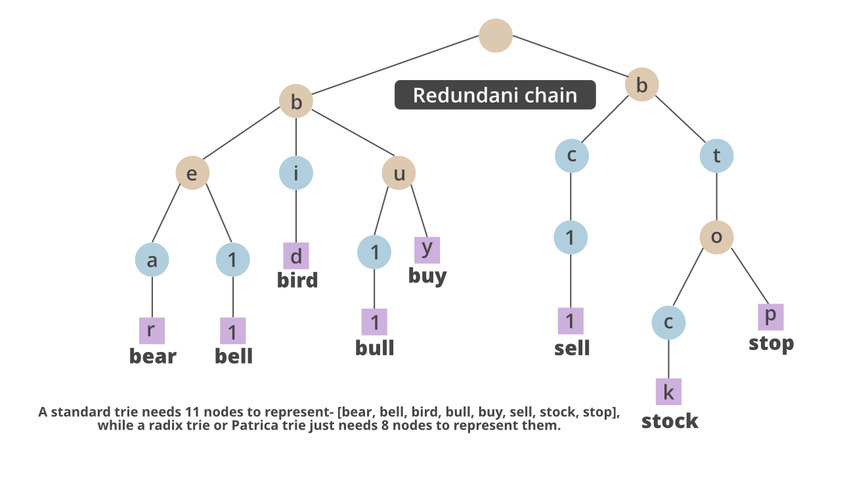Approach:

1. First, we simply create a class PatriciaTrieNode, in which we declare all the variables of the class.
2. Now we declare another PatriciaTest, where we build the PatriciaTest constructor
3. We declare functions like makeEmpty() or isEmpty() to check the Node’s status.
4. We will declare the function bit, which will help us to store the element in the node.
• We will first check if it’s length is not equal to max Bits.
• Then we write the code to get the ith bit of key k from left
5. Now we will write a function Boolean search which will help to find if the element is there in the  Node.
• Boolean search will take a number, which will help us search root node’
• PatriciaTrieNode search Node will search if the given data element is present or not
• If it is present return yes else no.
6. PatriciaTrieNode search is a function to search for an element.
• It will have two elements current and next Node.
• Next Node will be kept to the left child of the element t whereas the current Node is t.
• With the help of the while loop, we would check the next Node is greater than the current Node
• If satisfied, we would check if the current Node is equal to the next ‘Node.’
• Return next Node.
7. Now we would create a function insert PatriciaTrieNode
• Here we would declare current, parent, ‘LastNode’, ‘NewNode’.
• We would set the parameters like data, left Child, and right Child accordingly.
• We would also check the condition if we have already entered the same key
• If we did not enter it already we would store the key at a different variable
• Here we would set it to data, right child, left child, and so on
• If the parent matches the left child it is the NewNode or the right child becomes the NewNode
8. Now we would declare the main class
• We would declare the scanner
• We would also create an object for PatriciaTest
• We would declare a character
• Now we will declare the switch keyword
• This switch keyword can be accessed using a character.
• We can choose either to insert, search, empty, or to check if it is empty
• We can continue the loop according to the given input which satisfies the while.

Implementation:

Case 1

```Patricia Trie
Patricia Trie Operations
1. Insert
2. Search
3. Check Empty
4. Make Empty
1
Enter element to insert
10
Do you want to continue (Type y or n)
y
Patricia Trie Operations
1. Insert
2. Search
3. Check Empty
4. Make Empty
1
Enter element to insert
20
Do you want to continue (Type y or n)
y
Patricia Trie Operations
1. Insert
2. Search
3. Check Empty
4. Make Empty
1
Enter element to insert
30
Do you want to continue (Type y or n)
y
Patricia Trie Operations
1. Insert
2. Search
3. Check Empty
4. Make Empty
1
Enter element to insert
10
Do you want to continue (Type y or n)
y
Patricia Trie Operations
1. Insert
2. Search
3. Check Empty
4. Make Empty
2
Enter element to search
20
Search result : true
Do you want to continue (Type y or n)
y
Patricia Trie Operations
1. Insert
2. Search
3. Check Empty
4. Make Empty
4
Patricia Trie Cleared
Do you want to continue (Type y or n)
n```

Case 2

```Patricia Trie
Patricia Trie Operations
1. Insert
2. Search
3. Check Empty
4. Make Empty
3
Empty status : true
Do you want to continue (Type y or n)
y
Patricia Trie Operations
1. Insert
2. Search
3. Check Empty
4. Make Empty
1
Enter element to insert
5
Do you want to continue (Type y or n)
y
Patricia Trie Operations
1. Insert
2. Search
3. Check Empty
4. Make Empty
1
Enter element to insert
10
Do you want to continue (Type y or n)
y
Patricia Trie Operations
1. Insert
2. Search
3. Check Empty
4. Make Empty
1
Enter element to insert
15
Do you want to continue (Type y or n)
y
Patricia Trie Operations
1. Insert
2. Search
3. Check Empty
4. Make Empty
2
Enter element to search
10
Search result : true
Do you want to continue (Type y or n)
n```

Example

## Java

 `// Java Program to  implement Patricia trie`   `// Importing input output classes` `import` `java.io.*;` `// Importing Scanner class to display menu` `// or simply to take input from user` `import` `java.util.Scanner;`   `// Class 1` `// class PatriciaTrieNode is created` `// to obtained its elements` `class` `PatriciaTrieNode {` `    ``// Member variables of this class` `    ``// Declaring elements, number and data.` `    ``int` `number;` `    ``int` `data;`   `    ``// Two nodes are considered into action` `    ``// node1 -> left child and` `    ``// node2 -> right child` `    ``PatriciaTrieNode leftChild, rightChild;` `}`   `class`  `PatriciaTest {`   `    ``// Member variable of this class` `    ``// Declaring two elements` `    ``// Maxbits can help us to store elements in the Trie` `    ``// The root helps us to fix a global value.` `    ``private` `PatriciaTrieNode root;` `    ``private` `static` `final` `int` `MaxBits = ``10``;`   `    ``// Method 1` `    ``// PatriciaTrie where initially` `    ``// the root equals NULL` `    ``public`  `PatriciaTest() { root = ``null``; }`   `    ``// Method 2 - isEmpty()` `    ``// Method used to check if the function is empty as` `    ``// it returns true or false basing on the condition` `    ``public` `boolean` `isEmpty() { ``return` `root == ``null``; }`   `    ``// Method 3 - makeEmpty()` `    ``// Method  used to help in emptying the root` `    ``// of the Patricia Node` `    ``public` `void` `makeEmpty() { root = ``null``; }`   `    ``// Method 4 - bit()` `    ``// Declaring the function bit which performs a search` `    ``// operation in finding the bit which should be matched` `    ``// as input` `    ``private` `boolean` `bit(``int` `k, ``int` `i)` `    ``{` `        ``// Step 1 : Binary input is first converted to` `        ``// string as in strings its easy to match its` `        ``// corporate values` `        ``String binary = Integer.toString(k, ``2``);`   `        ``// Step2: Condition check while input length` `        ``// is not equal to the length of the maxbits` `        ``while` `(binary.length() != MaxBits)`   `            ``// Step 3: Keep adding the binary value` `            ``// until it gets the last number` `            ``binary = ``"0"` `+ binary;`   `        ``// Step 4: If the binary matches the desired value` `        ``//  needed, true will be returned` `        ``if` `(binary.charAt(i - ``1``) == ``'1'``)` `            ``return` `true``;`   `        ``// else we return false` `        ``return` `false``;` `    ``}`   `    ``// Method 5 - search()` `    ``public` `boolean` `search(``int` `k)` `    ``{` `        ``// Taking int num , as the half value of` `        ``// the of entered elements` `        ``int` `num = (``int``)(Math.log(k) / Math.log(``2``));`   `        ``// Condition check whether number` `        ``// is greater than maxBits` `        ``if` `(num > MaxBits) {` `            ``// Display message` `            ``// Print number has exceeded the limit` `            ``System.out.println(``"Exceeded the limit"``);`   `            ``// And return false` `            ``return` `false``;` `        ``}`   `        ``// Now when an element is created for the class` `        ``// named as 'searchNode'`   `        ``// This searches Node will go to the next` `        ``// search function` `        ``PatriciaTrieNode searchNode = search(root, k);`   `        ``// Now we will search the data element whether` `        ``// k is present in our node or not.`   `        ``// If it is present print true` `        ``// else print false` `        ``if` `(searchNode.data == k)` `            ``return` `true``;` `        ``else` `            ``return` `false``;` `    ``}`   `    ``// By now, search operation of` `    ``// PatriciaTrieNode class is declared` `    ``private` `PatriciaTrieNode search(PatriciaTrieNode t,` `                                    ``int` `k)` `    ``{`   `        ``// Now these are the currentNode and nextNode` `        ``PatriciaTrieNode currentNode, nextNode;`   `        ``// Step 1 : Now if the elements present in the t` `        ``// mode` `        ``//  are NULL,then NULL will be returned` `        ``if` `(t == ``null``) {` `            ``return` `null``;` `        ``}`   `        ``// Step 2: Now, considering the next node value to` `        ``// be the left child of the present variable t` `        ``nextNode = t.leftChild;`   `        ``// Step 3:  Next we keep the current node value` `        ``// to be "t"` `        ``currentNode = t;`   `        ``// Condition check` `        ``// Step 4: If the next node bitnumber is greater` `        ``// than the current numbers bitcode` `        ``while` `(nextNode.number > currentNode.number) {` `            ``// Step 5: Making the current Node as the next` `            ``// node`   `            ``// It is more like checking each` `            ``// as the next node becomes the current node` `            ``// Each time desired output won't be obtained` `            ``currentNode = nextNode;`   `            ``// Step 6: Putting this nextNode in the bitwise` `            ``// operator This method helps us to find whether` `            ``// it is LeftChild or Right Child` `            ``nextNode = (bit(k, nextNode.number))` `                           ``? nextNode.rightChild` `                           ``: nextNode.leftChild;` `        ``}` `        ``// Step 7: Now we return the next Node..` `        ``return` `nextNode;` `    ``}`   `    ``// Method 6 - insert()` `    ``// Inserting the value element inside PatriciaTrieNode` `    ``public` `void` `insert(``int` `element)` `    ``{` `        ``// Num is the variable where the value entered by` `        ``// the user will be stored. This value will be` `        ``// helpful to calculate the search index as well` `        ``int` `num` `            ``= (``int``)(Math.log(element) / Math.log(``2``)) + ``1``;`   `        ``// Now taking num greater than maxBits, it can be` `        ``// said` `        ``//  that the PatriciaTrieNode is full` `        ``if` `(num > MaxBits) {` `            ``// This will print the statement that we are` `            ``// full`   `            ``// Display message` `            ``System.out.println(` `                ``"We are full, The number is too large"``);`   `            ``return``;` `        ``}`   `        ``// Now the root value becomes the value` `        ``// where the element gets inserted` `        ``root = insert(root, element);` `    ``}`   `    ``// Now defining a function insert of the class` `    ``// PatriciaTrieNode` `    ``private` `PatriciaTrieNode insert(PatriciaTrieNode t,` `                                    ``int` `element)` `    ``{`   `        ``// Here the praticiaNode will have current , parent` `        ``// It will also have lastNode and newNode` `        ``PatriciaTrieNode current = ``null``, parent, lastNode,` `                         ``newNode;` `        ``int` `i;`   `        ``// Here t equals null`   `        ``// Condition check` `        ``// If it equals null simply declare` `        ``// the following attributes` `        ``if` `(t == ``null``) {` `            ``t = ``new` `PatriciaTrieNode();`   `            ``// Number is initialized to be 0` `            ``t.number = ``0``;`   `            ``// Data of the t node should be` `            ``// the element number` `            ``t.data = element;`   `            ``// where as the child will be t and` `            ``t.leftChild = t;`   `            ``// Right child of the t will be made empty` `            ``// or be equal to null` `            ``t.rightChild = ``null``;`   `            ``// Return the data t` `            ``return` `t;` `        ``}`   `        ``// Now declaring the lastNode to be search` `        ``lastNode = search(t, element);`   `        ``// If we declare the last node to be` `        ``// a part of the search function.`   `        ``// Now  we can compare it with the data` `        ``// already present in the PatriciaTrieNode` `        ``// If we have the key already Present` `        ``if` `(element == lastNode.data) {` `            ``// Print the display message` `            ``System.out.println(``"Key already Present"``);`   `            ``// Return t` `            ``return` `t;` `        ``}`   `        ``// Iterating variable  from` `        ``// first element to last element` `        ``for` `(i = ``1``;` `             ``bit(element, i) == bit(lastNode.data, i); i++)`   `            ``// Keep current to the left Child` `            ``current = t.leftChild;`   `        ``// Parent is equal to t` `        ``parent = t;`   `        ``// Condition check` `        ``// Current number is greater than parent number` `        ``// And if current number is less than i` `        ``while` `(current.number > parent.number` `               ``&& current.number < i) {` `            ``// If parent is current` `            ``parent = current;`   `            ``// Now we will see whether the new node` `            ``// is more flexible to the rightChild` `            ``// or is it ore available to the left child` `            ``// using scope resolution operator` `            ``current = (bit(element, current.number))` `                          ``? current.rightChild` `                          ``: current.leftChild;` `        ``}`   `        ``// Now we are taking this as newnode` `        ``newNode = ``new` `PatriciaTrieNode();`   `        ``// If we take newnode of number as i` `        ``newNode.number = i;`   `        ``// Now taking data as element` `        ``newNode.data = element;`   `        ``// Now taking the leftchild as depending on the` `        ``// condition` `        ``// we fix it either to be current or newNode` `        ``newNode.leftChild` `            ``= bit(element, i) ? current : newNode;`   `        ``// Now again taking the condition we fix` `        ``// The right child either to be newNode or` `        ``// curentNode` `        ``newNode.rightChild` `            ``= bit(element, i) ? newNode : current;`   `        ``// If we take current and parent as left child are` `        ``// same We fix them to be newNode` `        ``if` `(current == parent.leftChild) {` `            ``parent.leftChild = newNode;` `        ``}` `        ``else` `{` `            ``// else we take the right child to be the` `            ``// newNode` `            ``parent.rightChild = newNode;` `        ``}` `        ``// we return the value to t` `        ``return` `t;` `    ``}` `}`   `// Main Class` `public` `class` `GFG {`   `    ``// Main driver method` `    ``public` `static` `void` `main(String[] args)` `    ``{` `        ``// Scanner class to take input choices from user` `        ``Scanner sc = ``new` `Scanner(System.in);`   `        ``// Declare the object of the PatriciaTest class` `        ``PatriciaTest pt = ``new`  `PatriciaTest();`   `        ``// Display message` `        ``System.out.println(``"Patricia Trie\n"``);`   `        ``// Declaring a variable 'ch' of character with help` `        ``// of this character we will be able to make choiced` `        ``char` `ch;`   `        ``// Do-while is used for switching operations` `        ``// using switch case`   `        ``// Do loop includes execution in the body` `        ``// which will execute once atleast as` `        ``// condition is checked at last` `        ``do` `{` `            ``// Display Messages` `            ``// Heading would be patricia Trie Operations` `            ``System.out.println(` `                ``"\n Patricia Trie Operations\n"``);`   `            ``// Menu` `            ``// These are the following options` `            ``// that we would keep in a Patricia Trie`   `            ``// (1) Inserting the element` `            ``System.out.println(``"1. Insert"``);`   `            ``// (2) searching the element` `            ``System.out.println(``"2. Search"``);`   `            ``// (3) Checking for The Trie to be empty` `            ``System.out.println(``"3. Check Empty"``);`   `            ``// (4) Making it empty` `            ``System.out.println(``"4. Make Empty"``);`   `            ``// Display message` `            ``// Reading the choice of the user` `            ``System.out.println(``"Make your choice"``);`   `            ``// Switch variable` `            ``int` `choice = sc.nextInt();`   `            ``// Switch case keyboard enables to decide the` `            ``// choice` `            ``switch` `(choice) {` `                ``// Case 1 : Insertion` `                ``// We would simply call the insert function` `                ``// And set the data` `            ``case` `1``:` `                ``System.out.println(` `                    ``"Enter element to insert"``);` `                ``pt.insert(sc.nextInt());` `                ``break``;`   `                ``// Case 2: Enter the element to search` `            ``case` `2``:`   `                ``// If we would find the data we would give` `                ``// necessary output If not we would return` `                ``// false Print and display` `                ``System.out.println(` `                    ``"Enter element to search"``);` `                ``System.out.println(` `                    ``"Search result:"` `                    ``+ pt.search(sc.nextInt()));` `                ``break``;`   `                ``// Case: 3` `            ``case` `3``:`   `                ``// This is to check if the Trie is empty` `                ``// Print and display` `                ``System.out.print(``"Empty status : "` `                                 ``+ pt.isEmpty());` `                ``break``;`   `                ``// Case 4  : Empty the patricia Trie`   `            ``case` `4``:`   `                ``// Print and display` `                ``System.out.println(``"Patricie Trie Cleared"``);`   `                ``//  Calling makeEmpty() to empty the Trie` `                ``pt.makeEmpty();` `                ``break``;`   `                ``// Default case for invalid entry` `            ``default``:`   `                ``// Print and display` `                ``System.out.println(``"Wrong entry\n"``);` `                ``break``;` `            ``}`   `            ``// Now if we wish to continue` `            ``// Then we would press y and continue` `            ``// If not we would simply exit from the blocks` `            ``System.out.println(` `                ``"\n Do you want to continue (Type y or n)\n"``);` `            ``ch = sc.next().charAt(``0``);` `        ``}`   `        ``// Condition in do-while loop` `        ``while` `(ch == ``'Y'` `|| ch == ``'y'``);` `    ``}` `}`

Output:

Case 1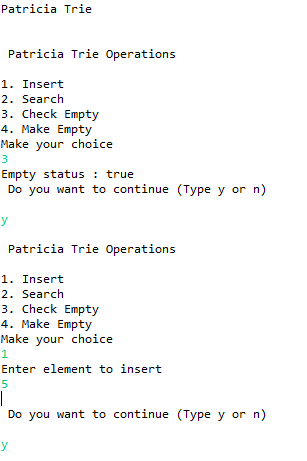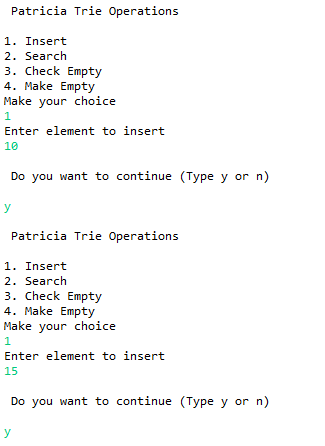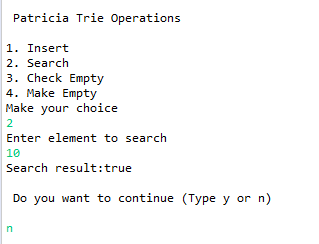Case 2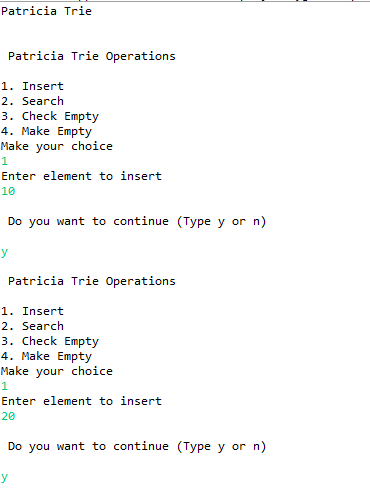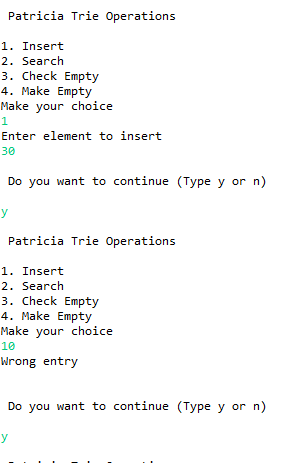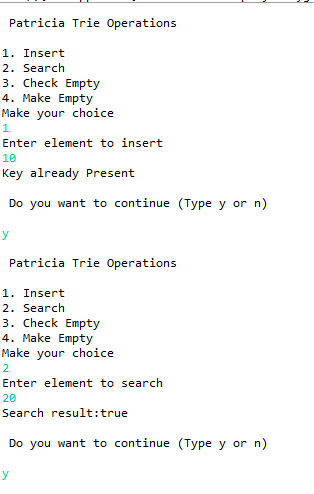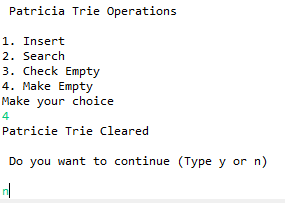My Personal Notes arrow_drop_up
Related Articles